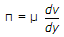# Civil Engineering - Hydraulics

Exercise : Hydraulics - Section 3
1.
For exerting a pressure of 4.8 kg/cm2, the depth of oil (specific gravity 0.8), should be
40 cm
41 cm
56 cm
60 cm
76 cm.
Explanation:
No answer description is available. Let's discuss.

2.
A cylindrical vessel 40 cm high is revolved about its vertical axis so that the water touches the bottom when it just spills out. If the radius of the cylinder is 5 cm, the angular velocity of rotation, is
none of these.
Explanation:
No answer description is available. Let's discuss.

3.
For the flow of liquid from an open ended tube (or nozzle) leading to the formation of spray of liquid drops, the number generally applied, is
Froude number
Weber number
Reynold number
Mach number.
Explanation:
No answer description is available. Let's discuss.

4.
In Newton's viscosity equation, the coefficient (μ) is known as coefficient of
viscosity
absolute viscosity
dynamic viscosity
simple viscosity
all the above.